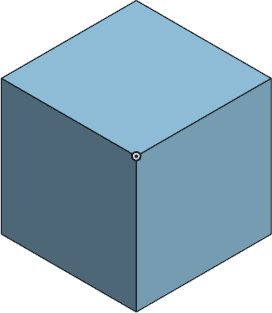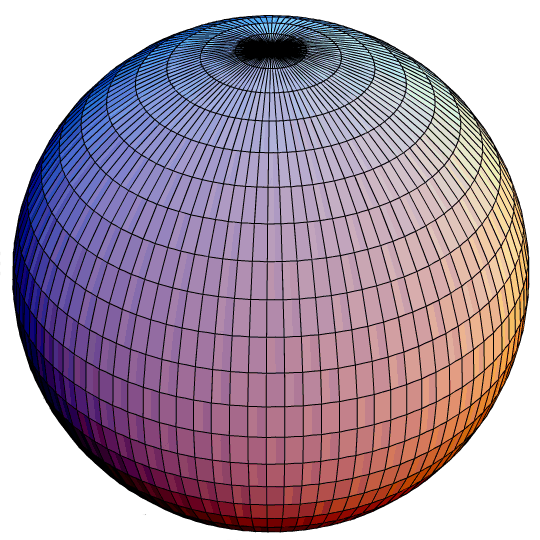# Questions About Volume (8 Things You Need To Know)

Volume is used throughout mathematics, physics, chemistry, and biology for a variety of applications.  However, there are some things you should know about volume, regardless of what you are using it for.

So, what do you need to know about volume?  Volume is never negative, but it can be zero if one dimension is zero. Volume can be less than surface area, but the units for them are different (square vs cubic). Surface area tells us how the volume of an object changes. The volume of an object can change if it is stretched or deformed.

Of course, we can also use antiderivatives in calculus to find the volume of objects in 3D space.  We can also optimize for the volume of an object for a given surface area to increase efficiency in manufacturing.

In this article, we’ll answer some common questions about volume, including how it relates to surface area for some common objects.  We’ll also go through some examples to make the concepts clear.

Let’s get started.

## Questions About Volume (8 Things You Need To Know)

Some of the most common questions about volume are those about the relationship between volume and surface area of common shapes (such as spheres and cubes).

However, before we get to that, let’s answer some more basic questions about volume – for example, can it be zero or negative?

### Can Volume Be Zero?

Volume can be zero.  This happens when at least one dimension of an object has a length of zero.

For example, a cylinder with zero height would have a volume of 0.  This is true regardless of the radius of the circular base, since:

• Vcylinder = πR2H
• Vcylinder = πR2(0)
• Vcylinder = 0A cylinder will have a volume of zero if its height is zero (a flat circle in 3D space).

In this case, the cylinder would be a flat circle in 3D space.

Similarly, a cube with a side length of zero would have a volume of zero, since:

• VCube = S3
• VCube = 03
• VCube = 0A cube will have a volume of zero if its side length is zero. In that case, it is just a point in 3D space.

In this case, the cube would be a single point in a 3D space.

A sphere with a radius of zero would also have a volume of zero, since:

• VSphere = 4πR3 / 3
• VSphere = 4π(0)3 / 3
• VSphere = 0

Note that the volume of a sphere is a cubic function in the variable R.A sphere will have a volume of zero if its radius is zero. In that case, it is just a point in 3D space.

In this case, the sphere would be a single point in a 3D space.

### Can Volume Be Negative?

Volume cannot be negative.  No matter the shape of the object in 3D space, its volume will either be zero (as noted above) or positive.

One good way to visualize this is to think of the volume of an object as the amount of water that an object will hold.  An object will hold at least 0 gallons of water; it will never hold a negative amount of water.

Another good way to see that volume cannot be negative is to consider the volume of a rectangular box.  Its volume is given by the formula:

• VBox = (Length)*(Width)*(Height)

In order for this volume to be negative, we would need at least one of the dimensions (length, width, or height) of the box to be negative.

However, this is impossible, since length is nonnegative by definition: distance is the absolute value of the straight-line distance between two points in 2D or 3D space.

Remember that to find the distance between two points (x1, y1) and (x2, y2) in 2D space, we take the positive square root:

• D = √((x2 – x1)2 + (y2 – y1)2)

Note that this formula comes from the Pythagorean Theorem, where the hypotenuse of the right triangle is the straight line segment between the two points (x1, y1) and (x2, y2).

We can extend this idea to three dimensions to find the distance between two points (x1, y1, z1) and (x2, y2, z2) in 2D space:

• D = √((x2 – x1)2 + (y2 – y1)2+ (z2 – z1)2)

Finally, remember that the density of an object is given by the formula

• Density = Mass / Volume

Since mass is always positive, we know that volume must be positive.  Otherwise, we could get a negative density, which would imply that an object would float in any material, including water and air!

### Can Volume Be Less Than Surface Area?

Volume can be less than surface area in some cases.  However, we must remember that we are comparing values with different units when we compare volume and surface area.

#### Example 1: Volume Of A Cube Less Than Surface Area Of A Cube

For example, let’s consider a cube with side length S.  The formulas for volume and surface area are given by:

• VCube = S3
• SACube = 6S2  [since there are 6 cube faces, each of which is a square with side length S and area S2]

So, to find out when the volume of the cube is less than the surface area of the cube, we need to solve the following inequality:

• VCube< SACube
• S3 < 6S2
• S3 – 6S2 < 0
• S2(S – 6) < 0

Since S2 is always positive for S > 0, we must have S – 6 < 0 or S < 6 to solve the inequality.

So, the volume of a cube is less than the surface area of the cube whenever the side length is less than 6.

#### Example 2: Volume Of A Sphere Less Than Surface Area Of A Sphere

For example, let’s consider a sphere with radius R.  The formulas for volume and surface area are given by:

• VSphere = 4πR3 / 3
• SASphere = 4πR2

So, to find out when the volume of the sphere is less than the surface area of the sphere, we need to solve the following inequality:

• VSphere< SASphere
• 4πR3 / 3 < 4πR2
• (4πR3 / 3) – 4πR2 < 0
• (4πR2 / 3)(R – 3) < 0

Since 4πR2 / 3is always positive for R > 0, we must have R – 3 < 0 or R < 3 to solve the inequality.

So, the volume of a sphere is less than the surface area of the sphere whenever the radius is less than 3.

### How Does Surface Area Relate To Volume?

For shapes like spheres and cubes, the surface area tells us how the volume of an object changes.  For a sphere, the surface area of the object is equal to the rate of change of the volume, or in calculus terms:

• VSphere’(R) = SASphere(R)  [surface area of a sphere is the derivative of the volume of the sphere]

For example, define the volume function of a sphere as a function of the variable R (radius) as:

• VSphere(R) = 4πR3 / 3

Then the derivative of the volume of a cube, with respect to the variable S (side length) is:

• VSphere’(R) = 3(4πR2 / 3)  [by the power rule for derivatives in calculus]
• VSphere’(R) = 4πR2 [3 cancels]
• SASphere(R) = 4πR2

This tells us that the surface area of the sphere tells us how the volume of the sphere changes as we increase (or decrease) the radius.

### Surface Area To Volume Ratios

We can also find out how surface area relates to volume by finding the ratio between the two for various objects, including spheres and cubes.

#### Surface Area To Volume Ratio Of A Cube

Remember from earlier that the volume and surface area of a cube are given by

• VCube = S3
• SACube = 6S2

To find the surface area to volume ratio of a cube, we simply divide the two and simplify:

• SACube / VCube
• =6S2 / S3
• =6 / S

Note: this gives us another way to find out when the volume of a cube is less than the surface area of a cube.  If the surface area to volume ratio is greater than 1, then the volume is less than the surface area, since:

• SACube / VCube > 1
• SACube > VCube

We can use the formula we calculated and simplified to find:

• 6 / S > 1
• 6 > S

This matches up with what we found earlier: the volume of a cube is less than the surface area of the cube when the side length S is less than 6.

#### Surface Area To Volume Ratio Of A Sphere

Remember from earlier that the volume and surface area of a sphere are given by

• VSphere = 4πR3 / 3
• SASphere = 4πR2

To find the surface area to volume ratio of a sphere, we simply divide the two and simplify:

• SASphere / VSphere
• 4πR2 / (4πR3 / 3)
• =3 / R

Note: this gives us another way to find out when the volume of a sphere is less than the surface area of a sphere.  If the surface area to volume ratio is greater than 1, then the volume is less than the surface area, since:

• SASphere / VSphere > 1
• SASphere > VSphere

We can use the formula we calculated and simplified to find:

• 3 / R > 1
• 3 > R

This matches up with what we found earlier: the volume of a sphere is less than the surface area of the sphere when the radius R is less than 3.

### Does Volume Change?

The volume of an object can change if the object is deformed or stretched in some way.  Think about a balloon as an example.

If you take an empty balloon, it does not contain any water.  However, if you put it on a hose and turn on the hose, it begins to fill with water.If you fill up a balloon with water or air, its volume changes, since the material stretches and is deformed.

As the balloon fills with water, the material stretches.  This deformation leads to a larger volume of water inside the balloon.

The same thing occurs when you fill a balloon with air.  However, there is a limit: whether you use water or air, the balloon will only be able to stretch so far before it bursts.

This is because the thickness of the balloon will only support so much weight or pressure of water before it ruptures.

## Conclusion

Now you know the answers to some common questions about volume.  You also know how surface area relates to volume for common objects like spheres and cubes.